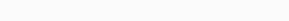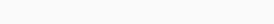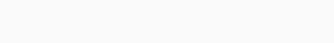# L2 and L1 as Regularization

In this lesson, you are going to learn what L2 and L1 functions are as Regularization also what regularization is actually. And also how it helps to prevent from overfitting.

## What is regularization?As in the above image the error of validation rate is not much decreasing as the training rate as iteration is increasing. Here regularization comes to the picture.

Regularization is a method by which we reduce overfitting or variance of neural network by decreasing the complexity. Complexities in our model may create the problem to evaluate even though its training results shows a good result. Therefore the regularization is a very important term to add in the loss function. It helps the model to give better result on new data. The implementation of regularization is very easy. Just add a term to the loss function that will take care for big weights.

## What actually L1 and L2 is?

The normalization vector is the foundation of L1 and L2.Here it is called 1-norm which is L1Here it is called 2-norm which is L2

## L1 regularization

The term which is going to add with loss function is simply the summation of weights magnitude with some regularization factor lambda (λ).

The L1 term isThen,

Cost function = Loss function + ( λ)* L1 term

## L2 regularization

The term which is going to add with loss function is simply the summation of squared weights with some regularization factor lambda (λ).

The L2 term isThen,

Cost function = Loss function + ( λ)* L2 term.